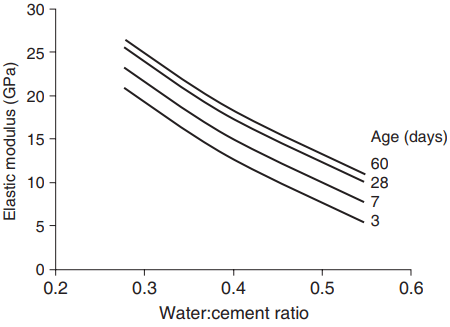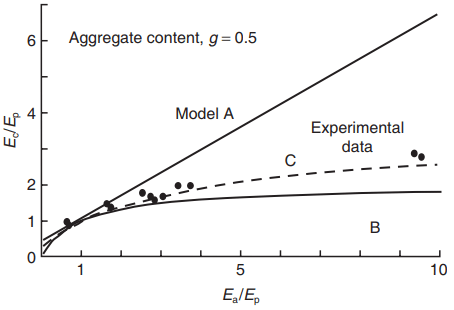# Stress–strain Behaviour  of Concrete

## Elasticity of Hardened Cement Paste

HCP has a near linear compressive stress–strain relationship for most of its range and therefore a modulus of elasticity can readily be determined from stress–strain data. Water-saturated pastes generally have a slightly higher modulus than dried pastes, indicating that some of the load is carried by the water in the pores.

Nevertheless, the skeletal lattice of the paste carries most of the load, and the elastic response is governed by the lattice properties. As might therefore be expected, the elastic modulus (Ep) is highly dependent on the capillary porosity (pc); the relationship has been found to be of the form:

Ep = Eg(1 – pc)3  …….(1)

where Eg is the modulus when pc = 0, i.e. it represents the modulus of elasticity of the gel itself.

This expression is a similar to the strength of the paste, and therefore it is to be expected that the same factors will influence both strength and modulus. This is indeed the case; for example, Fig. 1 shows that a decreasing water:cement ratio and increasing age both increase the elastic modulus.Fig. 1 Effect of water:cement ratio and age on theelastic modulus of hardened cement paste (after Hirsch,1962).

## Models for Concrete Behaviour

Concrete is, of course, a composite multiphase material, and its elastic behaviour will depend on the elastic properties of the individual phases – unhydrated cement, cement gel, water, coarse and fine aggregate and their relative proportions and geometrical arrangements. The real material is too complex for rigorous analysis, but if it is considered as a two-phase composite consisting of HCP and aggregate, then analysis becomes possible and instructive. Models for the behaviour of concrete require the following:

1. The property values for the phases; in this simple analysis, three are sufficient:
• the elastic modulus of the aggregate, Ea
• the elastic modulus of the HCP, Ep
• the volume concentration of the aggregate, g.
2. A suitable geometrical arrangement of the phases; three possibilities are shown in Fig. 2. All the models consist of unit cubes. Models A and B have the phases arranged as adjacent layers, the difference being that in A the two phases are in parallel, and therefore undergo the same strain, whereas in B the phases are in series and are therefore subjected to the same stress. Model C has the aggregate set within the paste such that its height and base area are both equal to √g, thus complying with the volume requirements. This intuitively is more satisfactory in that it bears a greater resemblance to concrete.

Analysis of the models is not intended to give any detail of the actual distribution of stresses and strains within concrete, but to predict average or overall behaviour. Three further assumptions are necessary:

1. The applied stress remains uniaxial and compressive throughout the model.
2. The effects of lateral continuity between the layers can be ignored.
3. Any local bond failure or crushing does not contribute to the deformation.

Model A – Phases in Parallel

Strain compatibility. The strain in the concrete, ec is equal to the strain in the aggregate, ea, and the paste, εp, i.e.

εc = εa = εp  …….(2)

Equilibrium. The total force is the sum of the forces on each of the phases. Expressed in terms of stresses and areas this gives:

σc.1 = σa.g + σp.(1 – g) …….(3)

Constitutive relations. Both of the phases and the concrete are elastic, hence:

σc = εc.Ec     σa = εa.Ea  and σp = εp.   …….(4)

where Ec, Ea and Ep are the elastic moduli of the concrete, aggregate and paste, respectively. Substituting into equation (3) from equation (4) gives:

εc.Ec = εa.Ea.g + εp.Ep.(1 – g) and hence, from equation (2)

Ec = Ea.g + Ep.(1 – g) ……..(5)

Model B – Phases in Series

Equilibrium. The forces and hence the stresses (since the forces act on equal areas) in both phases and the composite are equal, i.e.

σc = σa = σp  …….(6)

Strains. The total displacement is the sum of the displacements in each of the phases; expressed in terms of strain this gives:

εc = εa.g + εp.(1 – g) …….(7)

Substituting from equations (4) and (6) into (7) and rearranging gives:

1/Ec = g/Ea + (1 – g)/Ep ……..(8)

Model C – Combined

This is a combination of two layers of HCP alone in series with a third layer of HCP and aggregate in parallel, as in model A. Repetition of the above two analyses with substitution of the appropriate geometry and combination gives:

1/Ec = (1 – √g)/Ep + √g/(Ea.g + Ep[1 – √g]) …….(9)Fig. 3 The effect of volume concentration of aggregate on the elastic modulus of concrete calculated from thesimple two-phase models of Fig. 2.

Figure 3 shows the predicted results of equations 5, 8 and 9 from the three models for varying aggregate concentrations, with Ep < Ea as is normally the case. Models A and B give upper and lower bounds, respectively, to the concrete modulus, with model C, not surprisingly, giving intermediate values.Fig. 4 Prediction of the elastic modulus ofconcrete (Ec) from the moduli of the cement paste (Ep)and the aggregate (Ea) for 50% volume concentrationof the aggregate.

The effect of aggregate stiffness is shown in non-dimensional form in Fig. 4, on which some typical experimental results are also plotted. It is clear that for concrete in which Ea/Ep is near 1, e.g. with low-modulus lightweight aggregates, all three models give a reasonable fit, but for normal aggregates that are stiffer than the paste – i.e. Ea/Ep > 1 – model C is preferable.

## Measured Stress–strain Behaviour of Concrete

The stress–strain behaviour of both HCP and aggregate is substantially linear over most of the range up to a maximum. However, that of the composite concrete, although showing intermediate stiffness as predicted from the above analysis, is markedly non-linear over much of its length, as shown in Fig. 20.15a.

Furthermore, successive unloading/ loading cycles to stress levels below ultimate show substantial, but diminishing, hysteresis loops, and residual strains at zero load, as in Fig. 20.15b. The explanation for this behaviour lies in the contribution of microcracking to the overall concrete strain, i.e. assumption (3) in the above analysis is invalid.

As the transition zone between the aggregate and the HCP or mortar is a region of relative weakness, and in fact some microcracks will be present in this zone even before loading. The number and width of these will depend on such factors as the bleeding characteristics of the concrete immediately after placing and the amount of drying or thermal shrinkage. As the stress level increases, these cracks will increase in length, width and number, thereby making a progressively increasing contribution to the overall strain, resulting in non-linear behaviour.

Cracking eventually leads to complete breakdown and failure, therefore we will postpone more detailed discussion of cracking until the next chapter. Subsequent cycles of loading will not tend to produce or propagate as many cracks as the initial loading, provided the stress levels of the first or previous cycles are not exceeded. This explains the diminishing size of the hysteresis loops shown in Fig. 20.15b.

## Elastic Modulus of Concrete

The non-linear stress–strain curve of concrete means that a number of different elastic modulus values can be defined. These include the slope of the tangent to the curve at any point (giving the tangent modulus, A or B in Fig. 20.15b) or the slope of the line between the origin and a point on the curve (giving the secant modulus, C in Fig. 20.15b). A typical test involves loading to a working stress, say 40% of ultimate, and measuring the corresponding strain.

Cylindrical or prism specimens are usually used, loaded longitudinally, and with a length at least twice the lateral dimension. Strain measurements are usually taken over the central section of the specimen to avoid end effects.

To minimise hysteresis effects, the specimens are normally subjected to a few cycles of loading before the strain readings are taken over a load cycle lasting about five minutes. It is usual to calculate the secant modulus from these readings. This test is often called the static test – and the resulting modulus the static modulus – to distinguish it from the dynamic modulus test.

The elastic modulus increases with age and decreasing water:cement ratio of the concrete, for the reasons outlined above and, as with paste, these two factors combine to give an increase of modulus with compressive strength, but with progressively smaller increases at higher strength.

However, there is no simple relationship between strength and modulus since, as we have seen, the aggregate modulus and its volumetric concentration, which can vary at constant concrete strength, also have an effect. The modulus should therefore be determined experimentally if its value is required with any certainty.

This is not always possible, and estimates are often needed, e.g. early in the structural design process; for example the Eurocode 2 (BS EN 1992) gives a table of values for concrete with quartzite aggregates and compressive strengths in the range 20 to 110 MPa, which have the relationships:

Ec = 10fcube 0.31 and

Ec = 11fcyl 0.3

where Ec is the secant modulus of elasticity between zero and 40% of the ultimate stress; fcube is the mean cube compressive strength; and fcyl is the mean cylinder compressive strength.

For concrete with limestone and sandstone aggregates the values derived from these expressions should be reduced by 10 and 30%, respectively, and for basalt aggregates they should be increased by 20%.

## Poisson’s Ratio

The Poisson’s ratio of water-saturated cement paste varies between 0.25 and 0.3; on drying it reduces to about 0.2. It seems to be largely independent of the water:cement ratio, age and strength. For concrete, the addition of aggregate again modifies the behaviour, lower values being obtained with increasing aggregate content. For most concrete, values lie within the range 0.17 – 0.2.